C++ 虚函数表解析

# C++ 虚函数表解析

C++中的虚函数的作用主要是实现了多态的机制。关于多态，简而言之就是用父类型别的指针指向其子类的实例，然后通过父类的指针调用实际子类的成员函数。这种技术可以让父类的指针有“多种形态”，这是一种泛型技术。所谓泛型技术，说白了就是试图使用不变的代码来实现可变的算法。比如：模板技术，RTTI技术，虚函数技术，要么是试图做到在编译时决议，要么试图做到运行时决议。

#### 虚函数表

```class Base {
public:
virtual void f() { cout << "Base::f" << endl; }
virtual void g() { cout << "Base::g" << endl; }
virtual void h() { cout << "Base::h" << endl; }

};```

```typedef void(*Fun)(void);

Base b;

Fun pFun = NULL;

cout << "虚函数表地址：" << (int*)(&b) << endl;
cout << "虚函数表 — 第一个函数地址：" << (int*)*(int*)(&b) << endl;

// Invoke the first virtual function
pFun = (Fun)*((int*)*(int*)(&b));
pFun();```

Base::f

```(Fun)*((int*)*(int*)(&b)+0);  // Base::f()
(Fun)*((int*)*(int*)(&b)+1);  // Base::g()
(Fun)*((int*)*(int*)(&b)+2);  // Base::h()```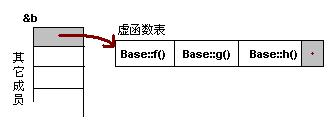#### 一般继承（无虚函数覆盖）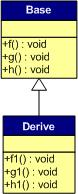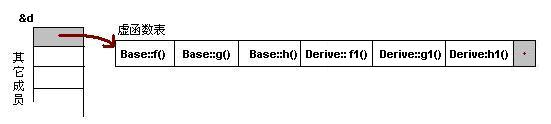1）虚函数按照其声明顺序放于表中。
2）父类的虚函数在子类的虚函数前面。

#### 一般继承（有虚函数覆盖）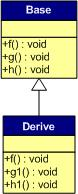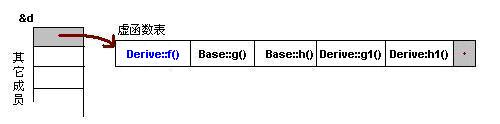1）覆盖的f()函数被放到了虚表中原来父类虚函数的位置。
2）没有被覆盖的函数依旧。

```Base *b = new Derive();

b->f();```

#### 多重继承（无虚函数覆盖）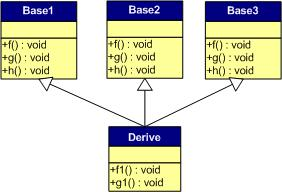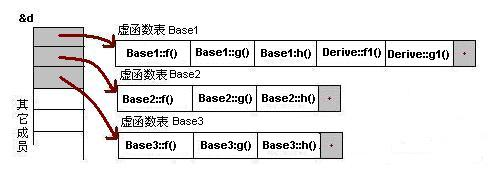1） 每个父类都有自己的虚表。
2） 子类的成员函数被放到了第一个父类的表中。（所谓的第一个父类是按照声明顺序来判断的）

#### 多重继承（有虚函数覆盖）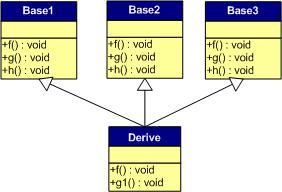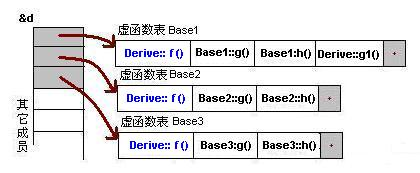```Derive d;
Base1 *b1 = &d;
Base2 *b2 = &d;
Base3 *b3 = &d;
b1->f(); //Derive::f()
b2->f(); //Derive::f()
b3->f(); //Derive::f()

b1->g(); //Base1::g()
b2->g(); //Base2::g()
b3->g(); //Base3::g()```

#### 安全性

##### 一、通过父类型的指针访问子类自己的虚函数

```Base1 *b1 = new Derive();
b1->f1();  //编译出错```

##### 二、访问non-public的虚函数

```class Base {
private:
virtual void f() { cout << "Base::f" << endl; }

};

class Derive : public Base{

};

typedef void(*Fun)(void);

void main() {
Derive d;
Fun  pFun = (Fun)*((int*)*(int*)(&d)+0);
pFun();
}```

#### 结束语

C++这门语言是一门Magic的语言，对于程序员来说，我们似乎永远摸不清楚这门语言背着我们在干了什么。需要熟悉这门语言，我们就必需要了解C++里面的那些东西，需要去了解C++中那些危险的东西。不然，这是一种搬起石头砸自己脚的编程语言。

##### 附录 二：例程

```#include <iostream>
using namespace std;

class Base1 {
public:
virtual void f() { cout << "Base1::f" << endl; }
virtual void g() { cout << "Base1::g" << endl; }
virtual void h() { cout << "Base1::h" << endl; }

};

class Base2 {
public:
virtual void f() { cout << "Base2::f" << endl; }
virtual void g() { cout << "Base2::g" << endl; }
virtual void h() { cout << "Base2::h" << endl; }
};

class Base3 {
public:
virtual void f() { cout << "Base3::f" << endl; }
virtual void g() { cout << "Base3::g" << endl; }
virtual void h() { cout << "Base3::h" << endl; }
};

class Derive : public Base1, public Base2, public Base3 {
public:
virtual void f() { cout << "Derive::f" << endl; }
virtual void g1() { cout << "Derive::g1" << endl; }
};

typedef void(*Fun)(void);

int main()
{
Fun pFun = NULL;

Derive d;
int** pVtab = (int**)&d;

//Base1's vtable
//pFun = (Fun)*((int*)*(int*)((int*)&d+0)+0);
pFun = (Fun)pVtab;
pFun();

//pFun = (Fun)*((int*)*(int*)((int*)&d+0)+1);
pFun = (Fun)pVtab;
pFun();

//pFun = (Fun)*((int*)*(int*)((int*)&d+0)+2);
pFun = (Fun)pVtab;
pFun();

//Derive's vtable
//pFun = (Fun)*((int*)*(int*)((int*)&d+0)+3);
pFun = (Fun)pVtab;
pFun();

//The tail of the vtable
pFun = (Fun)pVtab;
cout<<pFun<<endl;

//Base2's vtable
//pFun = (Fun)*((int*)*(int*)((int*)&d+1)+0);
pFun = (Fun)pVtab;
pFun();

//pFun = (Fun)*((int*)*(int*)((int*)&d+1)+1);
pFun = (Fun)pVtab;
pFun();

pFun = (Fun)pVtab;
pFun();

//The tail of the vtable
pFun = (Fun)pVtab;
cout<<pFun<<endl;

//Base3's vtable
//pFun = (Fun)*((int*)*(int*)((int*)&d+1)+0);
pFun = (Fun)pVtab;
pFun();

//pFun = (Fun)*((int*)*(int*)((int*)&d+1)+1);
pFun = (Fun)pVtab;
pFun();

pFun = (Fun)pVtab;
pFun();

//The tail of the vtable
pFun = (Fun)pVtab;
cout<<pFun<<endl;

return 0;
}```

(全文完)（转载本站文章请注明作者和出处 酷 壳 – CoolShell ，请勿用于任何商业用途）

——=== 访问 酷壳404页面 寻找遗失儿童。 ===——(28 人打了分，平均分： 4.39 )Loading...

## 《C++ 虚函数表解析》的相关评论

1.sunshinewong说道：

您这篇文章里的安全性部分的一、通过父类型的指针访问子类自己的虚函数，是不是说法不严谨。若按照您文章中说的，这种说法应该是不正确的，若是纯虚函数则就正确了。还望斧正！

2.wtz说道：

cout << "虚函数表地址：" << (int*)(&b) << endl;
这里明显有问题，这应该是虚函数表指针的地址，*(int*)(&b) 这样才是函数表的地址；
(int*)*(int*)(&b)，这个应该是指向虚函数表第一个元素(元素的值是虚函数地址)的指针，*(int*)*(int*)(&b)这才是第一个虚函数的地址

1.李班说道：

我觉得没问题.看看数组名退化成指针时和数组第一个元素的地址.函数名的字段可以代表一个函数指针

1.hhhhhhhh说道：

我觉得是虚函数表指针的地址，在类的内存分布，第一个是一个指针，指向v-table，并不是指向v-table的首元素，而是指向这个数组，所以是指向数组的指针，对其求地址，求的自然是虚函数表指针的地址。

1.JachinYang说道：

cout << “虚函数表地址：” << (int)(&b) << endl;这里确实表述有问题，首先我们实例化了一个对象b，那么存放b的内存空间的起始位置处应该存放的是指向虚函数表的指针，所以要想访问虚函数表的地址就需要解引用 cout << “虚函数表地址：” << *(int)(&b) << endl;

3.wtz说道：

看这篇文章的人注意，博主没写清楚，实际上对象的内存起始空间存放的是虚函数表的地址值，但不等同于说对象的起始地址就是虚函数表的地址；
博主如果说明虚函数表指针这个概念，应该会更容易让人理解。

1.JachinYang说道：

确实是这样

4.jiang说道：

第二张代码图里面 这行
pFun = (Fun)*((int*)*(int*)(&b));
好像不对，应该改成
pFun = (Fun)*((int**)*(int**)(&b));

我用的是mac上的clang编译器。

5.hawkcoder说道：

@jiang说的对，在Mac OS上是64位，而楼主用的是32位机，这会导致上述代码在64位系统运行失败。
*(int*)(&b)表示将b的地址转换成指向int型的指针，然后再取出其指针的值，这个值是32位int型。而在MAC系统64位中，取出的值应该要是64位才对，所以此时不能用*(int*)(&b) 而应该用*(long*)(&b)或者用一个指向指针的类型来转换比如*(int**)(&b),*(void**)(&b))…

6.土土哥说道：

有个问题，就是在子类已经覆盖了父类的虚函数的情况下，为什么子类还是可以调用“被覆盖”的父类的虚函数呢？
代码如下：

#include

using namespace std;

class Base {
public:
virtual void func() {
cout<<"Base func\n";
}
};

class Son : public Base {
public:
void func() {
Base::func();
cout<func();
delete b;
return 0;
}

输出：
Base func
Son func

1.李班说道：

你可以看看193页,可以静态的使用父类的开放的实体.而虚函数是动态的晚绑定.

1.李班说道：

深入探索c++对象模型…

7.Pounds说道：

@土土哥
这是C++提供的一个回避虚函数的机制
通过加作用域（正如你所尝试的），使得函数在编译时就绑定。
你也可以查看 C++ primer 5th P539页

8.Pounds说道：

有个问题就是如果子类没有覆写的虚函数真的会保存在后面么？
再保存次没有覆写的地址没意义啊，都指向同一个地方。
而且用visual studio ，在debug里显示vtable一直只有固定个数，和
博主图解有点出入哈？:-D
请教一下。

9.albumcover说道：

如@wtz所说，博主的代码说明有误。修改如下：

```cout << "对象地址：" << &b << endl; cout << "虚函数表地址：" << (int*)*(int*)(&b) << endl; cout << "虚函数表 — 第一个函数地址：" << (int*)*(int*)*(int*)(&b) << endl;```

``` ```

```// Invoke the first virtual function pFun = (Fun)*((int*)*(int*)(&b)); cout << "虚函数表 — 第一个函数地址(via pFun)：" << (int *)pFun << endl; ```

10.fengfengdiandian说道：

我使用vs2013、vs2017和GCC 6.3版本，虚函数表第一个地址是虚析构函数的，第二个地址才是对应的虚函数的地址

11.Y先森0.0说道：

这句话：‘在有虚函数的类的实例中这个表被分配在了这个实例的内存中‘，虚函数表不是存在于类中的吗？然后类的所有实例对象共享这个类的虚函数表

12.wahaha说道：

实在不明白，大家都指明了错误，而博主却不修改错误。虽然博主的文章很好。

1.JachinYang说道：

确实错了 但文章写的也确实好。

13.刘曦光说道：

64位机器代码，编译命令 g++ derive.cpp
#include

using namespace std;

class Base1 {
public:
virtual void f() { cout << “Base1:f” << endl;}
virtual void g() { cout << “Base1::g” << endl;}
};

class Base2 {
public:
virtual void f() { cout << “Base2:f” << endl;}
virtual void g() { cout << “Base2::g” << endl;}
};

class Base3 {
public:
virtual void f() { cout << “Base3:f” << endl;}
virtual void g() { cout << “Base3::g” << endl;}
};

class Derive : public Base1, public Base2, public Base3 {
public:
virtual void f() { cout << “Derive::f” << endl;}
virtual void g1() { cout << “Derive::g1” << endl;}
};

typedef void(*Func)(void);

int main() {
Func pFun = NULL;
Derive d;
long pVtab = (long)&d;

```// first virtual func table pFun = (Func)pVtab; pFun();```

``` pFun = (Func)pVtab; pFun(); pFun = (Func)pVtab; pFun(); pFun = (Func)pVtab; pFun(); pFun = (Func)pVtab; pFun(); pFun = (Func)pVtab; pFun(); pFun = (Func)pVtab; pFun(); ```

```return 0; ```

}

14.qinbei说道：

作者使用的机器应该是32位的机器，64位的机器应该使用long,以下代码再64位ubuntu18.04可以使用。
``` #include using namespace std;```

``` class Base { public: virtual void f(){ cout << "Base::f" << endl;} virtual void g(){ cout << "Base::g" << endl;} virtual void h(){ cout << "Base::h" << endl;} }; int main(int argc, char const argv[]) { typedef void(Fun) (void); Base b; Fun pfun; cout << "class address: \t" << &b << endl; cout << "virtual table address: \t" << (long)(&b) <<; endl; cout << "virtual table - first function address: \t" << (long)(long)(&b) << endl; pfun = (Fun)((long)(long)(&amp;b)); pfun(); return 0; ```

```} ```

15.aixi说道：

以前学虚函数表都是死记硬背，后来学C语言，读了一点Python虚拟机的代码，看到PyObject*，学习了PyTypeObject *ob_type这个结构之后，就有一种豁然开朗的感觉，原来虚函数表，虚函数表指针就是这么一种东西